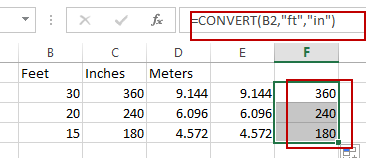## How to Auto Convert KB, MB, GB, PG, TB in Excel

This post will guide you how to convert value in KiB format to MiB, GiB, PiB and TiB automatically in Microsoft Excel. How do I convert size in bytes to KiB, MiB, GB, TiB and PiB in Excel 2013/2016/2019/365.

For example, assume that you have a list of data  which unit is bytes, and you wish to convert the unit of these values to KiB, MiB, GiB, TiB. You should know that the unit conversion rate is 1024 between each adjacent unit. So you can create a formula similar as the below:

``=B1/1024``

If you want to convert the unit from KiB to MiB, and you can type the following formula into a blank cell, then press Enter key.

``=B2/1024``

If you want to convert the unit from byte to MB, and you can use the following formula:

``=B1/1024/1024``

Or you can also use another formula based on the CONVERT function to convert a number from one measurement system to another.

The syntax of the Convert function is as below:

``=CONVERT (number, from_unit, to_unit)``

Where,

• Number – cell values that you want to convert its unit.
• From_unit – the source unit
• To_unit – the target unit

The prefixes of from/to unit should be Kibyte, Mibyte, Gibyte and Tibyte.

## 1. Convert KiB to MiB

To convert size in KiB to MiB, and you can use the following formula:

`` =CONVERT(B1,”kibyte”,”Mibyte”)``

## 2. Convert MiB to GiB

If you wish to convert the unit from MiB to GiB, and you can use the following convert formula:

`` =CONVERT(B2,”Mibyte”,”Gibyte”)``

## 3. Convert MiB to TiB

If the unit of your original data is MiB, and you wish to convert it as TiB, just use the below Convert formula:

`` =CONVERT(D2,”Mibyte”,”Tibyte”)``

## 4. Video: Auto Convert KiB, MiB, GiB, PiG, TiB in Excel

This video will demonstrate how to auto convert KiB, MiB, GiB, PiG, and TiB in Excel, including the specific conversions of KB to MiB, MiB to GiB, and MiB to TiB.

## BMI Calculation Formula In Ms Excel

You might have come across a task in which you were assigned to make BMI calculations of the supplied numbers, and you may be looking for an efficient approach to accomplish this process rather than doing BMI calculations manually, by which you can also complete that task, but ultimately you will end up exhausted.

But don’t worry; we’ve got your back and brought up a most accessible way to perform BMI calculations in a couple of seconds.

So, without further ado, let’s get started.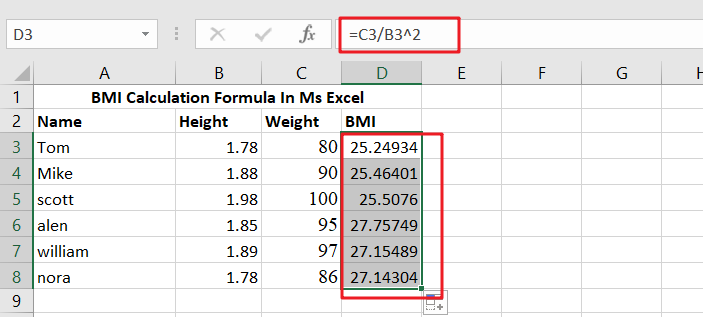## What Exactly Is BMI?

Body Mass Index is an abbreviation for Body Mass Index. It is a simple (and affordable) method of determining body fat based on height and weight. BMI is a screening technique that may determine if a person is underweight, overweight, or obese. BMI, on the other hand, is not a diagnostic tool.

## General Formula

The algorithm below may be used to compute BMI calculations.

`=weight/height^2`

## Explanation of Syntax

You must be aware of the Formula’s syntax to use it to complete your task.

• Division (/): This symbol divides values or integers.

## Summary

This tutorial will look at two different ways to compute BMI (Body Mass Index) in Excel. We first convert height and weight to metric units in the example worksheet before calculating BMI using a conventional metric method. In D3, the Formula is:

`=C3/B3^2`

It delivers an estimated BMI based on a G5 weight and an F5 height## Explanation

It is easier to grasp the application of the above Formula with the assistance of two examples. The first example demonstrates an effective method for calculating BMI (Body Mass Index) in Excel. The typical BMI formula is as follows:

`BMI = weight (kg) / height (m)2`

The second method employed here is to first convert height in inches and feet to meters, and weight in pounds to kilograms, before applying the conventional metric calculation for BMI. This allows for collecting size and weight, generally used units (in the United States), and displaying measured quantities utilized in the computation.

The fundamental difficulty, in this case, is that most Americans still use the US conventional measuring system to record height and weight rather than the metric system. As a result, the initial step is to record this data is regularly used units.

Then, in cell E12, we utilize the CONVERT function twice to compute height in meters:

`=CONVERT(B12,"ft","m")+CONVERT(C12,"in","m")`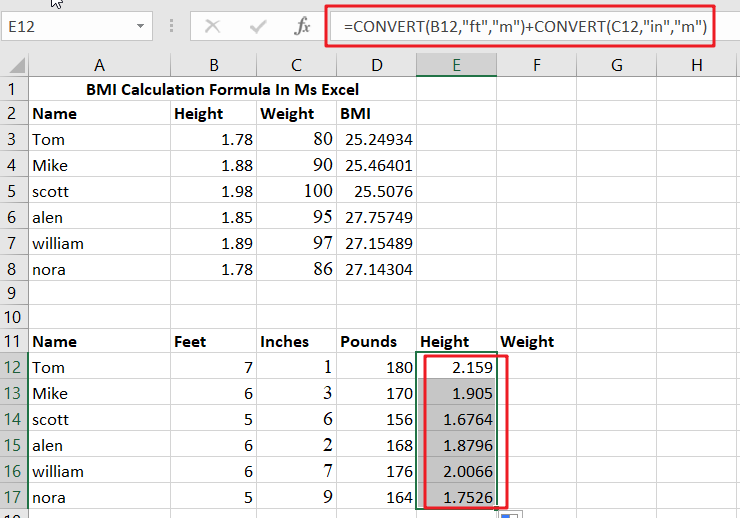The first CONVERT translates feet to meters as follows:

`=CONVERT(B12,"ft","m")/ convert feet to meters`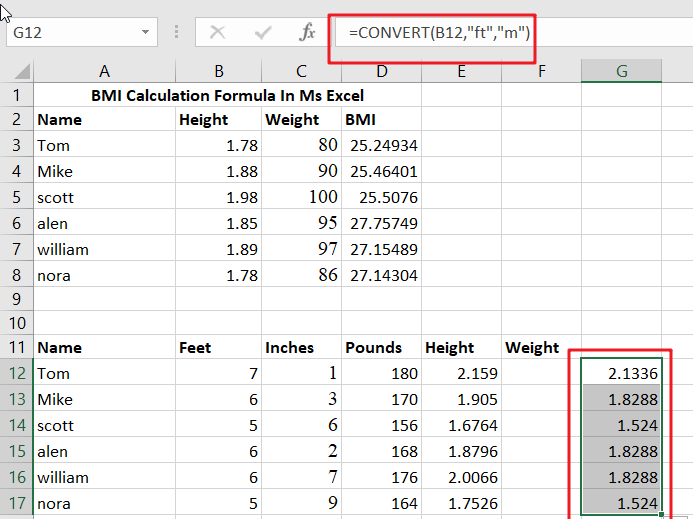The second translates inches to meters as follows:

`=CONVERT(C12,"in","m")/ converting inches to meters`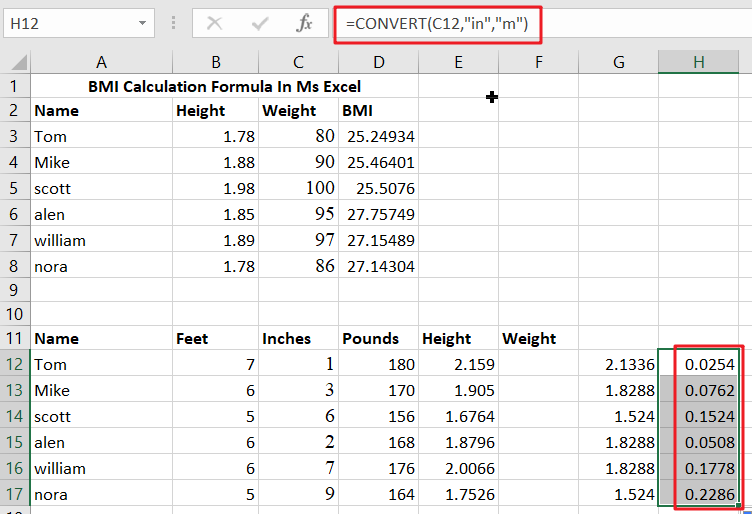The two numbers are then put together to obtain the total height in meters.

In cell F12, we use CONVERT once again to determine the weight in kilograms:

`=CONVERT(D12,"lbm","kg")/ converting pounds to kilograms`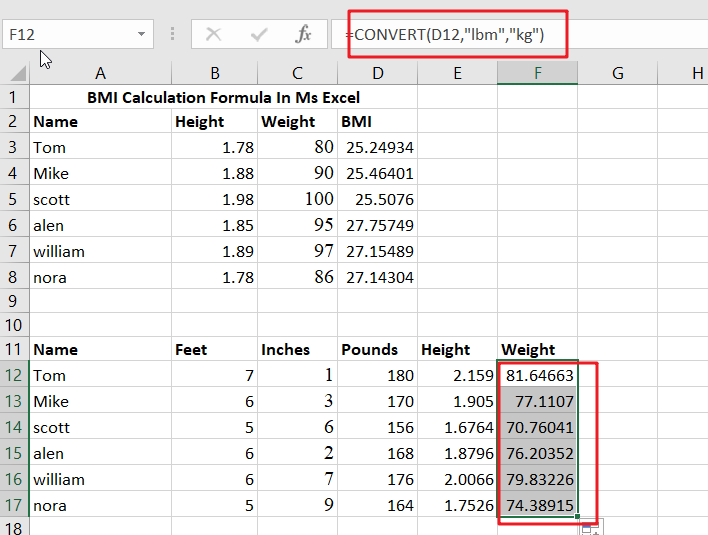Finally, we may use the usual BMI formula. In G12, the Formula is:

`=F12/E12^2/ compute BMI`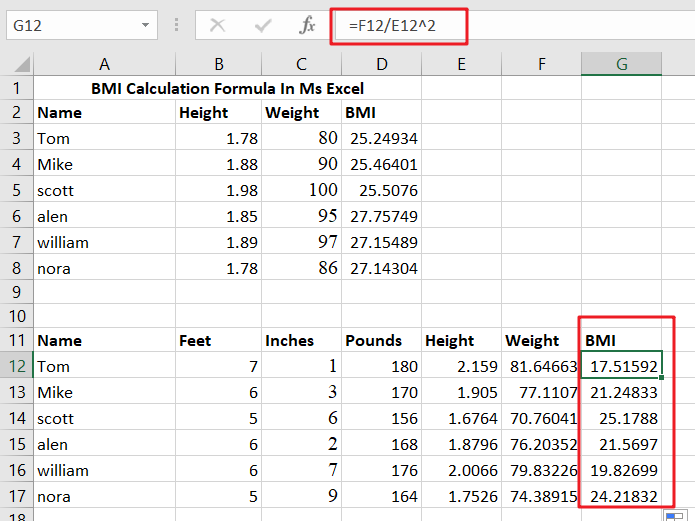We utilize Excel’s exponentiation operator, the caret () to square height.

In addition to the caret (), the POWER function may be used to raise to a power such as this:

`=F12/POWER(E12,2)`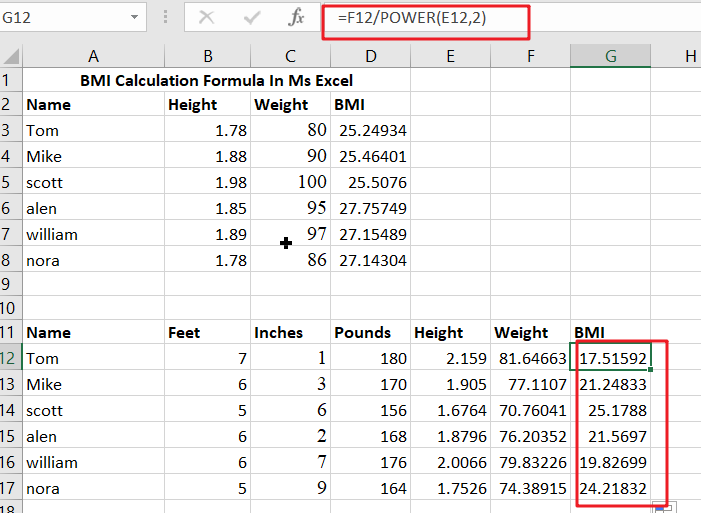## Alternatives

The formulae mentioned above can be simplified somewhat. We may use a single CONVERT function to determine the height, as seen below:

`=CONVERT(B12*12+C12,"in","m")`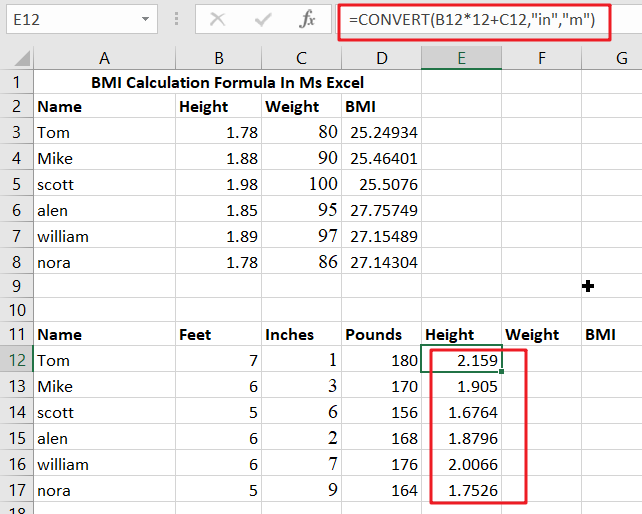To put it another way, we convert feet to inches immediately within the function parameter. This process occurs before the CONVERT function is called when Excel evaluates the calculation. It’s not as readable, but it’s more compact.

Similarly, we might use CONVERT to convert inches to feet as follows:

`=CONVERT(B12+C12/12,"ft","m")`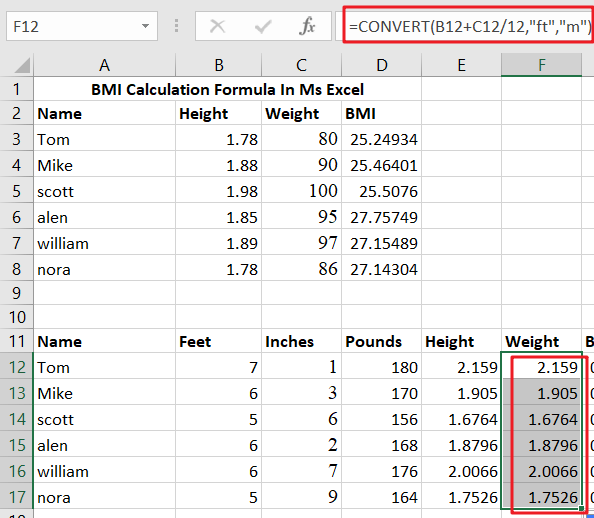The end outcome is the same as before. The critical feature is that you may nest additional calculations directly in a function parameter.

## How to Convert Ounces to Pounds or Grams in Excel

This post will guide you how to convert ounces to pounds or grams with formula in Excel. How do I convert pounds to ounces or grams in Excel 2013/2016.

## Convert Ounces to Pounds

Assuming that you have a list of data in range B1:B4, in which contain values with Ounces data, and you wish to convert these Ounces to pounds. How to do it. You can use an formula based on the CONVERT function to convert it quickly. Like this:

`=CONVERT(B2,"ozm","lbm")`

You need to type this formula into a blank cell such as: Cell C2, and press Enter key on your keyboard, and then drag the AutoFill handle down to other cells to apply this formula.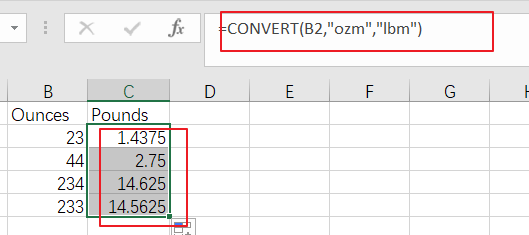## Convert Pounds to Ounces

If you want to convert your range of cells with Pounds data to Ounces, you can use the below formula based on the CONVERT function:

`=CONVERT(C2,"lbm","ozm")`

You need to type this formula into a blank cell such as: Cell D2, and press Enter key on your keyboard, and then drag the AutoFill handle down to other cells to apply this formula.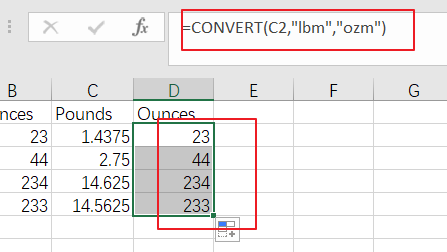## Convert Ounces to kg

If you want to convert kg into Ounces for you range B2:B5, just use the following formula:

`=CONVERT(B2,"ozm","kg")`

You need to type this formula into a blank cell such as: Cell E2, and press Enter key on your keyboard, and then drag the AutoFill handle down to other cells to apply this formula.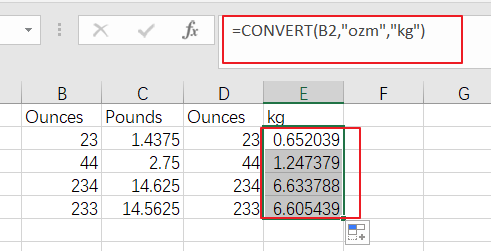## How to Convert Fahrenheit to Celsius or Kelvin in Microsoft Excel

This post will guide you how to Convert Temperatures among Fahrenheit, Celsius, and kelvin using a formula in Microsoft Excel. How do I convert Fahrenheit to Celsius in Excel 2013/2016. How to convert degrees Fahrenheit to degrees Celsius in Excel. The Fahrenheit unit is a measure of temperature primarily used in the United States, but most of countries in Europe are using Celsius Units for temperature. Sometimes you may be need to measure Fahrenheit and convert it to Celsius. This post will show you one method to convert it.

## Convert Fahrenheit to Celsius

Assuming that you have a list of data in range B1:B5 in which contain some temperature values and its unit is Fahrenheit. And you want to convert Fahrenheit to Celsius for those values in the current worksheet. You can easily use a built-in function called CONVERT to accomplish it. And this function can be used to convert between different units of measurement. The CONVERT function needs three arguments that you need to provide, the first argument is the value that you would like to convert, the second argument is the unit of measurement you are converting from, and the last argument is the unit of measurement of you are converting to. So you can use the following formula to convert your data from Fahrenheit unit to Celsius:

`=CONVERT(B1,"F","C")`

Type this formula into a blank cell, such as: C1, and press Enter key on your keyboard to complete the conversion. And then drag the AutoFill handle over to cell C5 to apply this formula.

Note: if you want to convert Celsius to Fahrenheit, just use the following formula based on the CONVERT function:

`=CONVERT(C1,"C","F")`

And if you want to Convert Fahrenheit to Kelvin, just using the following formula:

`=CONVERT(B1,"F","K")`

## How to Convert the Unit of Measurement for Cells in Excel

This post will guide you how to convert the unit of measurement from one unit to another in Excel. How do I convert various unit measurements in cells with a formula in Excel 2013/2016.

## Convert Unit of Measurement

Assuming that you have a list of data that contain numeric values, and you want to convert a measurement from one unit to anther, such as: from “inch” to “meter”. You can use the CONVERT function to convert the unit of measurement for cells. You just need to enter data and units into the following formula:

=CONVERT(number,”from_unit”,”to_unit”)

For example, you want to change the unit of measurement for cell C1 from inch to meter, you can use the following formula:

`=CONVERT(C1,”in”,”m”)`

Note: you need to enter in the approved abbreviated form of the original unit into the second parameter, such as: “in”, and then enter in the approved abbreviated form for “to_unit” in the third parameter.

You need enter this formula into a blank cell and press Enter key on your keyboard to apply this formula.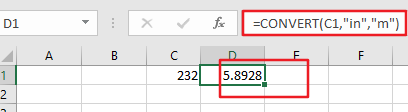Then you would see that the unit measurement has been converted.

## How to Convert Times in Excel

This post will guide you how to convert hours into minutes with a formula in Excel. How do I convert time to hours, minutes, or seconds or days in Excel.

## Convert between Time Units

If you want to find out how many hours there are in n days, or how many days are in n years in Excel, you can use a formula based on the CONVERT function to convert time between time units.

Convert Days to Hours

Assuming that you have a list of data in range B1:B5, in which contain day values, and if you want to convert all days to hours in a given range, you can use the CONVERT function to create the following formula to achieve it.

`=CONVERT(B2,"day","hr")`

Type this formula into a blank cell and press Enter key, and then drag the AutoFill Handle over to other cells to apply this formula.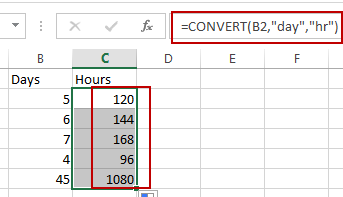Convert Hours to Days

Assuming that you have a list of data in range B1:B5, in which contain Hour values, and if you want to convert all hours to days in a given range, you can use the CONVERT function to create the following formula to achieve it.

`=CONVERT(B2,"hr","day")`

Type this formula into a blank cell and press Enter key, and then drag the AutoFill Handle over to other cells to apply this formula.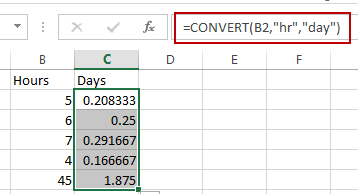Convert Years to Days

If you want to convert Years to days, just use the following formula:

`=CONVERT(B2,"yr","day")`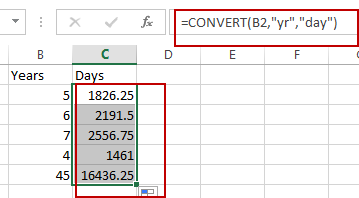Convert Hours to Minutes

If you want to convert Hours to Minutes, just use the following formula:

`=CONVERT(B2,"hr","mn")`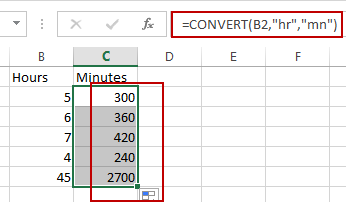## Convert measurements

This post will guide you how to convert feet to inches in Excel. How to convert feet to meters in Excel. How to convert fee to inches or meters with CONVERT function in Excel.

## Convert Feet to Inches with a Formula

If you want to convert feet to inches with a calculator, you need to multiply feet by 12. As one foot is equal to 12 inches. So you can use the following formula to convert the measurement unit from feet to inches:

`=B2 * 12`

Type this formula into cell C2, and press Enter key in your keyboard, and then drag the AutoFill Handle over other cells to apply this formula.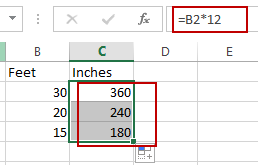If you want to convert feet to meters, you can multiply feet by 0.3048. And one foot is equal to 0.3048 meters. So you can convert feet to meters with the following formula:

`=B2*0.3048`

Type this formula into cell D2, and then press Enter key.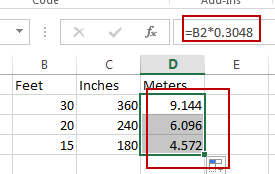## Convert Feet to Meters with Convert Function

You can also use a formula based on the CONVERT function to convert feet to meters in Excel. Just use the following formula:

`=CONVERT(B2,"ft","m")`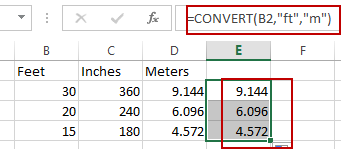If you want to convert feet to inches, you can use the following formula:

`=CONVERT(B2,"ft","in")`